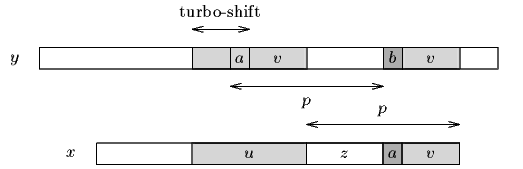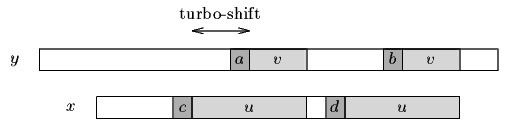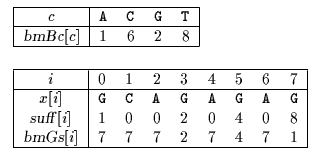Next: Apostolico-Giancarlo algorithm Up: ESMAJ Prev: Boyer-Moore algorithm

# Turbo-BM algorithm

Main features
• variant of the Boyer-Moore;
• no extra preprocessing needed with respect to the Boyer-Moore algorithm;
• constant extra space needed with respect to the Boyer-Moore algorithm;
• preprocessing phase in O(m+) time and space complexity;
• searching phase in O(n) time complexity;
• 2n text character comparisons in the worst case.
Description

The Turbo-BM algorithm is an amelioration of the Boyer-Moore algorithm. It needs no extra preprocessing and requires only a constant extra space with respect to the original Boyer-Moore algorithm. It consists in remembering the factor of the text that matched a suffix of the pattern during the last attempt (and only if a good-suffix shift was performed).it is possible to jump over this factor;it can enable to perform a turbo-shift.

A turbo-shift can occur if during the current attempt the suffix of the pattern that matches the text is shorter than the one remembered from the preceding attempt. In this case let us call u the remembered factor and v the suffix matched during the current attempt such that uzv is a suffix of x. Let a and b be the characters that cause the mismatch during the current attempt in the pattern and the text respectively. Then av is a suffix of x, and thus of u since |v| < |u|. The two characters a and b occur at distance p in the text, and the suffix of x of length |uzv| has a period of length p=|zv| since u is a border of uzv, thus it cannot overlap both occurrences of two different characters a and b, at distance p, in the text. The smallest shift possible has length |u|-|v|, which we call a turbo-shift (see figure 14.1).Figure 14.1. A turbo-shift can apply when |v|<|u|.

Still in the case where |v|<|u| if the length of the bad-character shift is larger than the length of the good-suffix shift and the length of the turbo-shift then the length of the actual shift must be greater or equal to |u|+1. Indeed, in this case the two characters c and d are different since we assumed that the previous shift was a good-suffix shift. (see figure 14.2)Figure 14.2.cd so they cannot be aligned with the same character in v.

Then a shift greater than the turbo-shift but smaller than |u|+1 would align c and d with a same character in v. Thus if this case the length of the actual shift must be at least equal to |u|+1.

The preprocessing phase can be performed in O(m+) time and space complexity. The searching phase is in O(n) time complexity. The number of text character comparisons performed by the Turbo-BM algorithm is bounded by 2n.

The C code

The functions preBmBc and preBmGs are given chapter Boyer-Moore algorithm.

In the TBM function, the variable u memorizes the length of the suffix matched during the previous attempt and the variable v memorizes the length of the suffix matched during the current attempt.

```void TBM(char *x, int m, char *y, int n) {
int bcShift, i, j, shift, u, v, turboShift,
bmGs[XSIZE], bmBc[ASIZE];

/* Preprocessing */
preBmGs(x, m, bmGs);
preBmBc(x, m, bmBc);

/* Searching */
j = u = 0;
shift = m;
while (j <= n - m) {
i = m - 1;
while (i >= 0 && x[i] == y[i + j]) {
--i;
if (u != 0 && i == m - 1 - shift)
i -= u;
}
if (i < 0) {
OUTPUT(j);
shift = bmGs;
u = m - shift;
}
else {
v = m - 1 - i;
turboShift = u - v;
bcShift = bmBc[y[i + j]] - m + 1 + i;
shift = MAX(turboShift, bcShift);
shift = MAX(shift, bmGs[i]);
if (shift == bmGs[i])
u = MIN(m - shift, v);
else {
if (turboShift < bcShift)
shift = MAX(shift, u + 1);
u = 0;
}
}
j += shift;
}
}

```
The example

Preprocessing phasebmBc and bmGs tables used by Turbo Boyer-Moore algorithm

Searching phase

References
• CROCHEMORE, M., 1997. Off-line serial exact string searching, in Pattern Matching Algorithms, ed. A. Apostolico and Z. Galil, Chapter 1, pp 1-53, Oxford University Press.
• CROCHEMORE, M., CZUMAJ A., GASIENIEC L., JAROMINEK S., LECROQ T., PLANDOWSKI W., RYTTER W., 1992, Deux méthodes pour accélérer l'algorithme de Boyer-Moore, in Théorie des Automates et Applications, Actes des 2e Journées Franco-Belges, D. Krob ed., Rouen, France, 1991, pp 45-63, PUR 176, Rouen, France.
• CROCHEMORE, M., CZUMAJ, A., GASIENIEC, L., JAROMINEK, S., LECROQ, T., PLANDOWSKI, W., RYTTER, W., 1994, Speeding up two string matching algorithms, Algorithmica 12(4/5):247-267.
• CROCHEMORE, M., RYTTER, W., 1994, Text Algorithms, Oxford University Press.
• LECROQ, T., 1992, Recherches de mot, Ph. D. Thesis, University of Orléans, France.
• LECROQ, T., 1995, Experimental results on string matching algorithms, Software - Practice & Experience 25(7):727-765.Next: Apostolico-Giancarlo algorithm Up: ESMAJ Prev: Boyer-Moore algorithm

e-mails: {Christian.Charras, Thierry.Lecroq }@laposte.net
Tue Jan 14 15:03:31 MET 1997